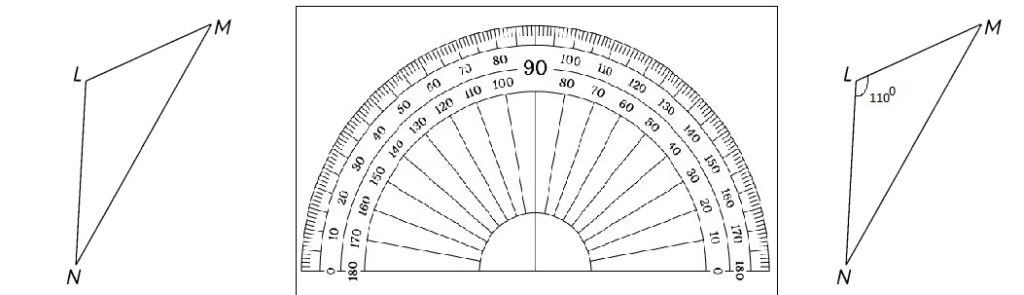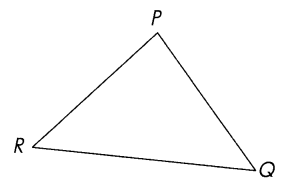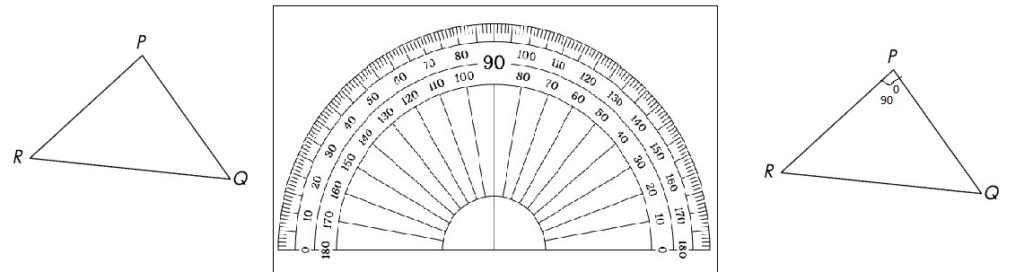# Math in Focus Grade 5 Chapter 13 Practice 1 Answer Key Classifying Triangles

This handy Math in Focus Grade 5 Workbook Answer Key Chapter 13 Practice 1 Classifying Triangles provides detailed solutions for the textbook questions.

## Math in Focus Grade 5 Chapter 13 Practice 1 Answer Key Classifying Triangles

Which of these triangles are equilateral, isosceles, or scalene? Use a centimeter ruler to find out.

Question 1.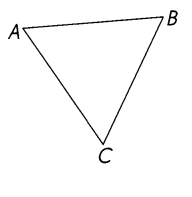Triangle ABC is a/an triangle.
__Equilateral_ triangle.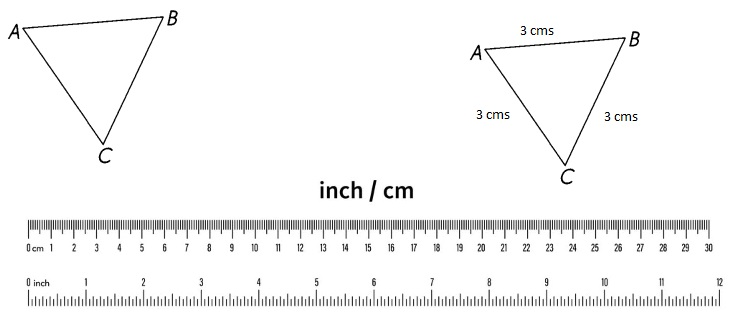Triangle ABC is an equilateral triangle,

Explanation:
Given triangle ABC is an equilateral triangle because all
given 3 sides are equal.

Question 2.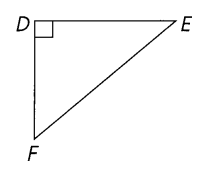Triangle DEF is a/an
_____ triangle.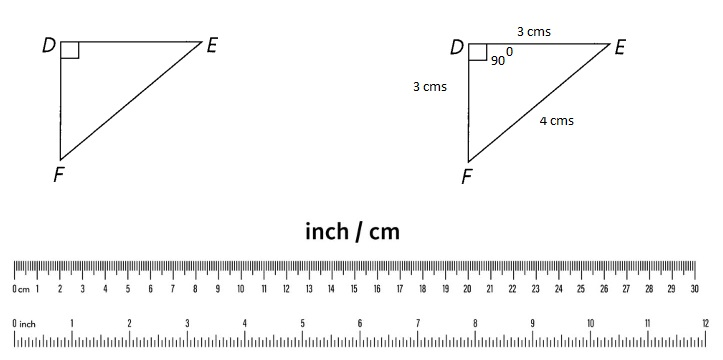Triangle DEF is an isosceles triangle,

Explanation:
Given triangle DEF is an isosceles triangle because
only 2 sides are equal and congurent.

Question 3.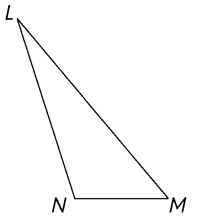Triangle LMN is a/an
_____ triangle.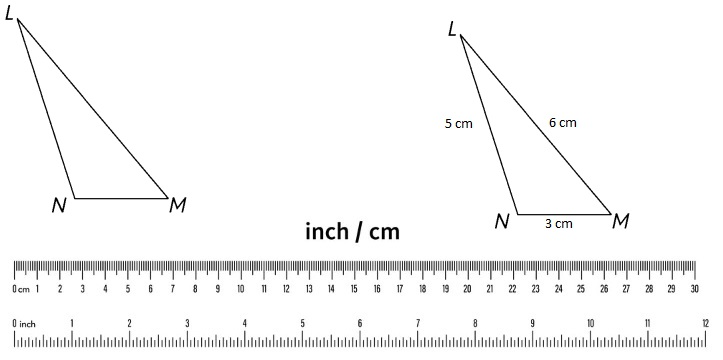Triangle LMN is an  scalene triangle,

Explanation:
Given triangle LMN is an scalene triangle because all
given 3 sides are not equal as LM ≠ MN ≠ LN.

Question 4.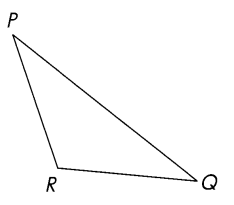Triangle PQR is a/an
___ triangle.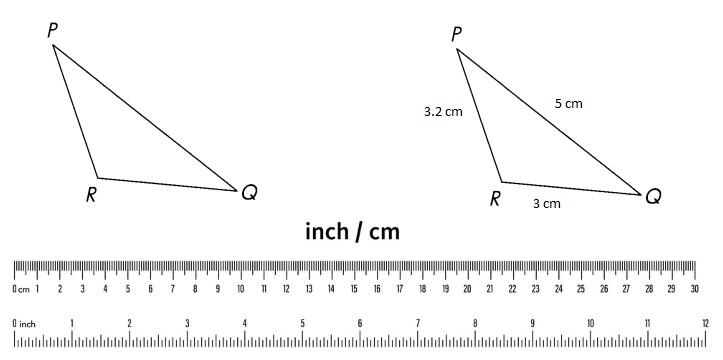Triangle PQR is a scalene triangle,

Explanation:
Given triangle PQR is a scalene triangle because all
given 3 sides are not equal as PQ ≠ PR ≠ RQ.

Which of these triangles are right, obtuse, or acute? Use a protractor to find out.

Question 5.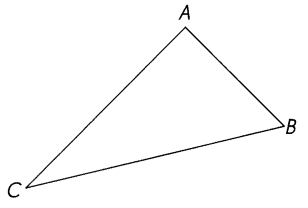Triangle ABC is a/an
____ triangle.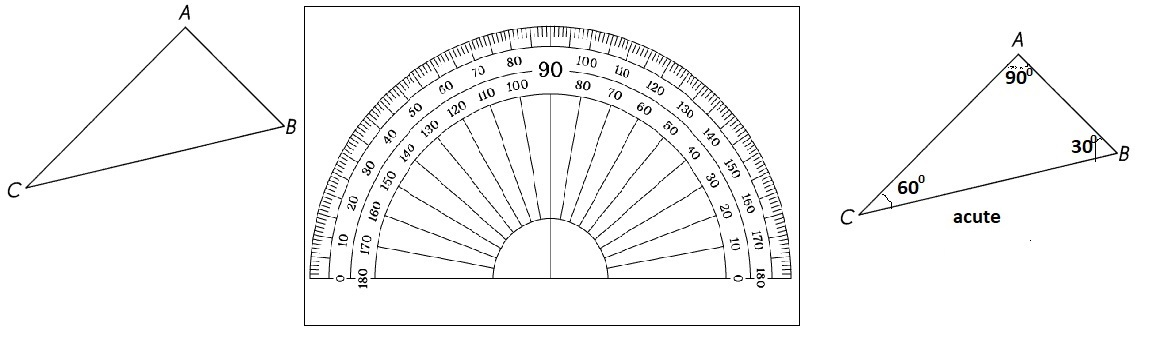Explanation:
Given triangle ABC is acute triangle, because angle B and C is measuring less than 900.

Question 6.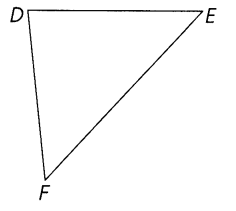Triangle DEF is a/an
____ triangle.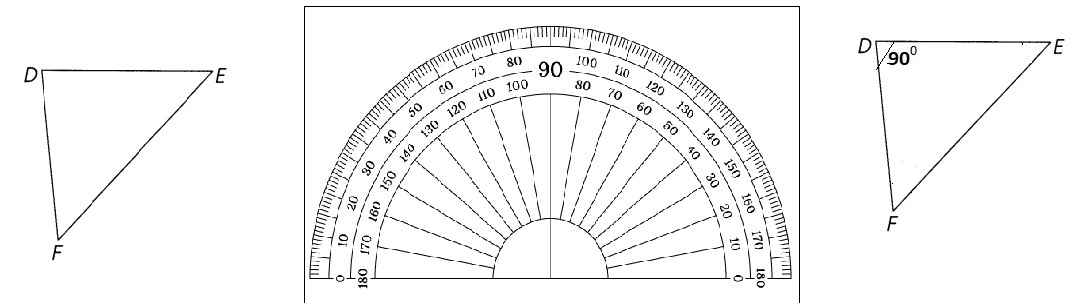Explanation:
Given triangle DEF is right triangle, because angle D is measuring 900.

Question 7.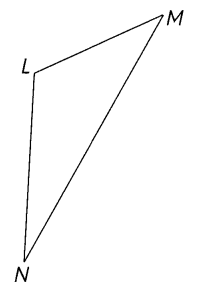Triangle LMN is a/an
____ triangle.